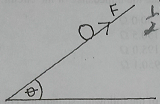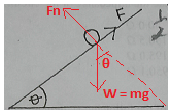mycollegehive
The diagram above illustrates a ball of mass m sliding dow...
waec-2020 ssce-exam-2020 waec-physics-2020 physics0The diagram above illustrates a ball of mass m sliding down a plank inclined at an angle of θ to the horizontal. The kinetic friction between the ball and the plank is F and acceleration of free fall is g

The normal force on the ball is

A. mgsinθ

B. mgtanθ

C. mgcosθ

D. mgcotθ

146 viewsShareFollowUniversity of Lagos Nigeria
31 August 2020School not set Nigeria
31 August 20200The normal force is the force that extends perpendicular (90o) to the surface.W is the weight of the ball and Fn is the normal force.

From the above diagram, resolving W to the the Fn (extended or broken line), yields: mgcosθShare

How to insert math formulas/equations### Related Tags

waec-2020

8 followers

273 questionsssce-exam-2020

6 followers

273 questionswaec-physics-2020

6 followers

48 questions